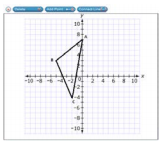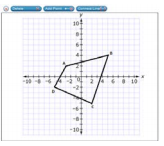# MAFS.8.G.1.3

Describe the effect of dilations, translations, rotations, and reflections on two-dimensional figures using coordinates.
General Information
Subject Area: Mathematics
Domain-Subdomain: Geometry
Cluster: Level 2: Basic Application of Skills & Concepts
Cluster: Understand congruence and similarity using physical models, transparencies, or geometry software. (Major Cluster) -

Clusters should not be sorted from Major to Supporting and then taught in that order. To do so would strip the coherence of the mathematical ideas and miss the opportunity to enhance the major work of the grade with the supporting clusters.

Date of Last Rating: 02/14
Status: State Board Approved
Assessed: Yes
Test Item Specifications

• Assessment Limits :
Coordinate values of ?? and ?? must be integers. The number of transformations should be no more than two. In items that require the student to draw a transformed figure using a dilation or a rotation, the center of the transformation must be given.
• Calculator :

Neutral

• Context :

Allowable

Sample Test Items (3)
• Test Item #: Sample Item 1
• Question: Triangle ABC is translated 5 units to the right to create triangle A’B’C’.Use the Connect Line tool to draw triangle A’B’C’.

• Difficulty: N/A
• Type: GRID: Graphic Response Item Display

• Test Item #: Sample Item 2
• Question:Use the Connect Line tool to draw quadrilateral A’B’C’D’.

• Difficulty: N/A
• Type: GRID: Graphic Response Item Display

• Test Item #: Sample Item 3
• Question:

A pentagon is shown.

The pentagon is translated 5 units to the left and then reflected over the x-axis.

Use the Connect Line tool to draw the pentagon after its transformations.

• Difficulty: N/A
• Type: GRID: Graphic Response Item Display

## Related Courses

This benchmark is part of these courses.
1205050: M/J Accelerated Mathematics Grade 7 (Specifically in versions: 2014 - 2015, 2015 - 2020, 2020 - 2022 (current), 2022 and beyond)
1205070: M/J Grade 8 Pre-Algebra (Specifically in versions: 2014 - 2015, 2015 - 2022 (current), 2022 and beyond)
1204000: M/J Foundational Skills in Mathematics 6-8 (Specifically in versions: 2014 - 2015, 2015 - 2022 (current), 2022 and beyond)
7812030: Access M/J Grade 8 Pre-Algebra (Specifically in versions: 2014 - 2015, 2015 - 2018, 2018 - 2019, 2019 and beyond (current))
7912115: Fundamental Explorations in Mathematics 2 (Specifically in versions: 2013 - 2015, 2015 - 2017 (course terminated))

## Related Access Points

Alternate version of this benchmark for students with significant cognitive disabilities.
MAFS.8.G.1.AP.3a: Dilate common polygons using graph paper and identifying the coordinates of the vertices.
MAFS.8.G.1.AP.3b: Given two figures on a coordinate plane, identify if the image is dilated, translated, rotated, or reflected.

## Related Resources

Vetted resources educators can use to teach the concepts and skills in this benchmark.

## Assessments

Sample 4 - Eighth Grade Math State Interim Assessment:

This is a State Interim Assessment for eighth grade.

Type: Assessment

Sample 3 - Eighth Grade Math State Interim Assessment:

This is a State Interim Assessment for eighth grade.

Type: Assessment

## Educational Software / Tool

Transformations Using Technology:

This virtual manipulative can be used to demonstrate and explore the effect of translation, rotation, and/or reflection on a variety of plane figures. A series of transformations can be explored to result in a specified final image.

Type: Educational Software / Tool

## Formative Assessments

Translation Coordinates:

Students are asked to translate two-dimensional figures in the coordinate plane and identify the coordinates of the vertices of the images.

Type: Formative Assessment

Rotation Coordinates:

Students are asked to rotate two-dimensional figures in the coordinate plane and identify the coordinates of the vertices of the images.

Type: Formative Assessment

Reflection Coordinates:

Students are asked to reflect two-dimensional figures in the coordinate plane and identify the coordinates of the vertices of the images.

Type: Formative Assessment

Dilation Coordinates:

Students are asked to dilate two-dimensional figures in the coordinate plane and identify the coordinates of the vertices of the images.

Type: Formative Assessment

## Lesson Plans

Coding Geometry Challenge #10 & 11:

This set of geometry challenges focuses on scaled drawings and area as students problem solve and think as they learn to code using block coding software.  Student will need to use their knowledge of the attributes of polygons and mathematical principals of geometry to accomplish the given challenges. The challenges start out fairly simple and move to more complex situations in which students can explore at their own pace or work as a team. Computer Science standards are seamlessly intertwined with the math standards while providing “Step it up!” and “Jump it up!” opportunities to increase rigor.

Type: Lesson Plan

Coding Geometry Challenge #23 & 24:

This set of geometry challenges focuses on using transformations to show similarity and congruence of polygons and circles. Students problem solve and think as they learn to code using block coding software.  Student will need to use their knowledge of the attributes of polygons and mathematical principals of geometry to accomplish the given challenges. The challenges start out fairly simple and move to more complex situations in which students can explore at their own pace or work as a team. Computer Science standards are seamlessly intertwined with the math standards while providing “Step it up!” and “Jump it up!” opportunities to increase rigor.

Type: Lesson Plan

Slide to the Left... Slide to the Right!:

Students will identify, review, and analyze transformations. They will demonstrate their understanding of transformations in the coordinate plane by creating original graphs of polygons and the images that result from specific transformations.

Type: Lesson Plan

Exploring Rotations with GeoGebra:

This lesson will help students understand the concept of a geometric rotation. The teacher/students will use a GeoGebra applet to derive the rules for rotating a point on the coordinate plane about the origin for a 90 degree, 180 degree, and a 270 degree counterclockwise rotation.

Type: Lesson Plan

Reflecting Reflections:

In this resource, students experiment with successive reflections of a triangle in a coordinate plane.

Point Reflection:

The purpose of this task is for students to apply a reflection to a single point. The standard asks students to apply rigid motions to lines, line segments, and angles. Although this problem only applies a reflection to a single point, it has high cognitive demand if the students are prompted to supply a picture. This is because the coordinates of the point (1000,2012) are very large. If students try to plot this point and the line of reflection on the usual x-y coordinate grid, then either the graph will be too big or else the point will lie so close to the line of reflection that it is not clear whether or not it lies on this line. A good picture requires a careful choice of the appropriate region in the plane and the corresponding labels. Moreover, reflections of lines, line segments, and angles are all found by reflecting individual points.

Triangle congruence with coordinates:

In this resource, students will decide how to use transformations in the coordinate plane to translate a triangle onto a congruent triangle. Exploratory examples are included to prompt analytical thinking.

## Student Center Activity

Students can practice answering mathematics questions on a variety of topics. With an account, students can save their work and send it to their teacher when complete.

Type: Student Center Activity

## Tutorial

Scaling Down a Triangle by Half:

This video demonstrates the effect of a dilation on the coordinates of a triangle.

Type: Tutorial

## Virtual Manipulative

Rotation of a Point:

This virtual manipulative is an interactive visual presentation of the rotation of a point around the origin of the coordinate system. The original point can be dragged to different positions and the angle of rotation can be changed with a 90° increment.

Type: Virtual Manipulative

## MFAS Formative Assessments

Dilation Coordinates:

Students are asked to dilate two-dimensional figures in the coordinate plane and identify the coordinates of the vertices of the images.

Reflection Coordinates:

Students are asked to reflect two-dimensional figures in the coordinate plane and identify the coordinates of the vertices of the images.

Rotation Coordinates:

Students are asked to rotate two-dimensional figures in the coordinate plane and identify the coordinates of the vertices of the images.

Translation Coordinates:

Students are asked to translate two-dimensional figures in the coordinate plane and identify the coordinates of the vertices of the images.

## Student Resources

Vetted resources students can use to learn the concepts and skills in this benchmark.

## Educational Software / Tool

Transformations Using Technology:

This virtual manipulative can be used to demonstrate and explore the effect of translation, rotation, and/or reflection on a variety of plane figures. A series of transformations can be explored to result in a specified final image.

Type: Educational Software / Tool

Reflecting Reflections:

In this resource, students experiment with successive reflections of a triangle in a coordinate plane.

Point Reflection:

The purpose of this task is for students to apply a reflection to a single point. The standard asks students to apply rigid motions to lines, line segments, and angles. Although this problem only applies a reflection to a single point, it has high cognitive demand if the students are prompted to supply a picture. This is because the coordinates of the point (1000,2012) are very large. If students try to plot this point and the line of reflection on the usual x-y coordinate grid, then either the graph will be too big or else the point will lie so close to the line of reflection that it is not clear whether or not it lies on this line. A good picture requires a careful choice of the appropriate region in the plane and the corresponding labels. Moreover, reflections of lines, line segments, and angles are all found by reflecting individual points.

Triangle congruence with coordinates:

In this resource, students will decide how to use transformations in the coordinate plane to translate a triangle onto a congruent triangle. Exploratory examples are included to prompt analytical thinking.

## Student Center Activity

Students can practice answering mathematics questions on a variety of topics. With an account, students can save their work and send it to their teacher when complete.

Type: Student Center Activity

## Tutorial

Scaling Down a Triangle by Half:

This video demonstrates the effect of a dilation on the coordinates of a triangle.

Type: Tutorial

## Virtual Manipulative

Rotation of a Point:

This virtual manipulative is an interactive visual presentation of the rotation of a point around the origin of the coordinate system. The original point can be dragged to different positions and the angle of rotation can be changed with a 90° increment.

Type: Virtual Manipulative

## Parent Resources

Vetted resources caregivers can use to help students learn the concepts and skills in this benchmark.

Reflecting Reflections:

In this resource, students experiment with successive reflections of a triangle in a coordinate plane.